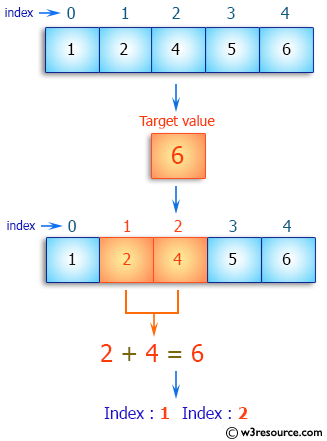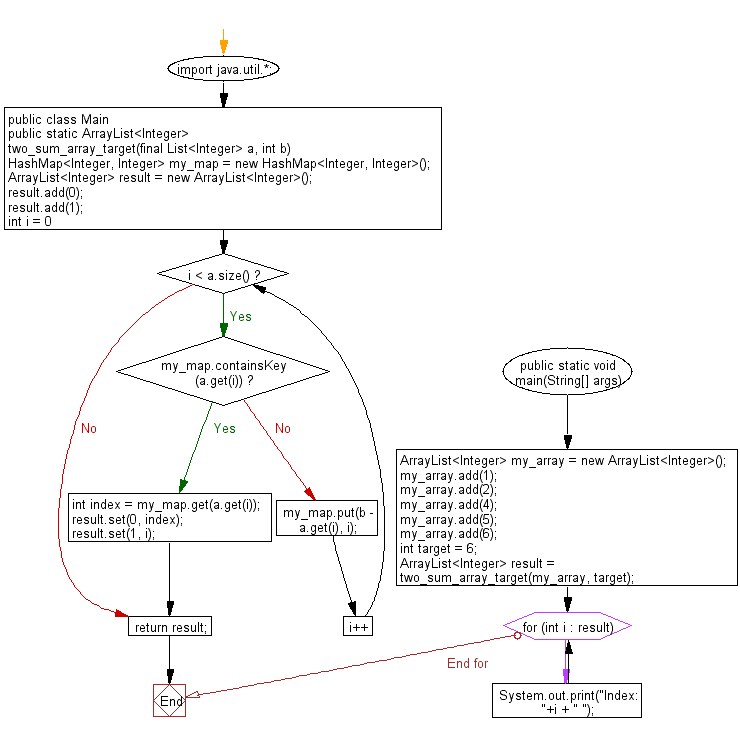﻿ Java: Sum of 2 values of an array which is equal to a value# Java Array Exercises: Find the sum of the two elements of a given array which is equal to a given integer

## Java Array: Exercise-35 with Solution

Write a Java program to find the sum of the two elements of a given array which is equal to a given integer.
Sample array: [1,2,4,5,6]
Target value: 6.

Pictorial Presentation:Sample Solution:

Java Code:

``````import java.util.*;
public class Exercise35
{
public static ArrayList<Integer> two_sum_array_target(final List<Integer> a, int b) {

HashMap<Integer, Integer> my_map = new HashMap<Integer, Integer>();
ArrayList<Integer> result = new ArrayList<Integer>();
for(int i = 0; i < a.size(); i++){
if(my_map.containsKey(a.get(i))){
int index = my_map.get(a.get(i));
result.set(0, index );
result.set(1, i );
break;
}
else{
my_map.put(b - a.get(i), i);
}
}

return result;
}

public static void main(String[] args){
ArrayList<Integer> my_array = new ArrayList<Integer>();
int target = 6;
ArrayList<Integer> result = two_sum_array_target(my_array, target);
for(int i : result)
System.out.print("Index: "+i + " ");
}
}
```
```

Sample Output:

```
Index: 1 Index: 2
```

Flowchart:Visualize Java code execution (Python Tutor):

Java Code Editor:

Improve this sample solution and post your code through Disqus

What is the difficulty level of this exercise?

Test your Programming skills with w3resource's quiz.

﻿

## Java: Tips of the Day

countOccurrences

Counts the occurrences of a value in an array.

Use Arrays.stream().filter().count() to count total number of values that equals the specified value.

```public static long countOccurrences(int[] numbers, int value) {
return Arrays.stream(numbers)
.filter(number -> number == value)
.count();
}
```

Ref: https://bit.ly/3kCAgLb